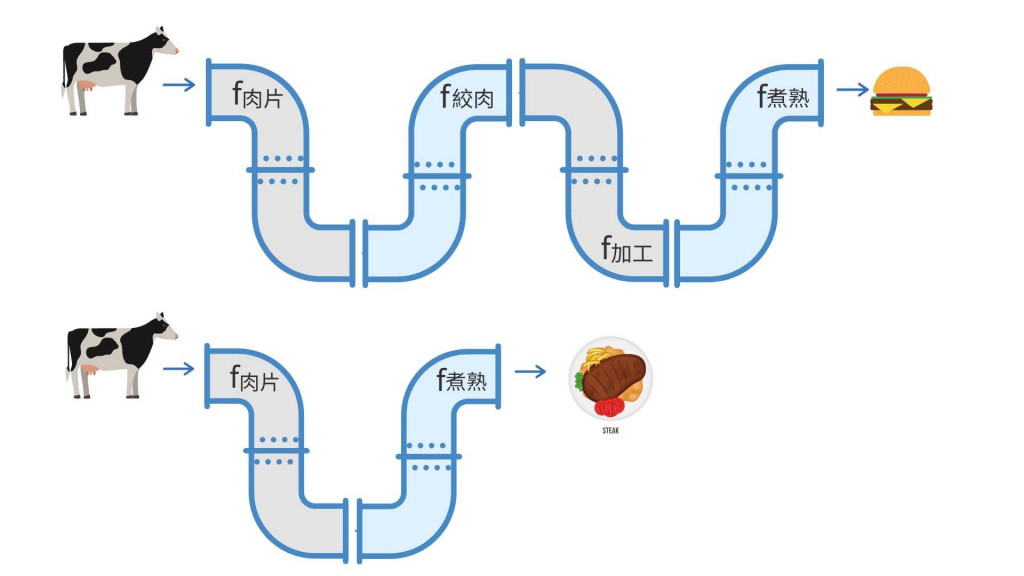#DAY 17
1
Software Development

## [補充] Ramda.js# What's Ramda?

A practical functional library for JavaScript programmers.

Ramda 是一個 Library ，可以把 Ramda 想成 functional programming 界的 lodash 或 underscore 。

# Why use Ramda?

Ramda 的 API 很豐富，而且大部分 API 也很直覺，不需要花太多時間理解。但你可能會覺得我用 loadash 就好，為何要多學一個？最大的差別在於

• Ramda 強調函式都是 pure 的。Immutability 跟不造成 side-effect 是他的設計理念，也就是說我們可以寫出更簡單優雅的程式碼
• 任何 API 都會自動 curry 化，這特性提供了程式碼相當大的彈性
• "Function first，Data last"

It's very easy to build functions as sequences of simpler functions, each of which transforms the data and passes it along to the next.## What Ramda looks like?

`_.函式名稱(函式, 資料)`

``````const _ = R;

const odd = x => x%2 === 1
const data = [3, 5, 6];
_.filter(odd, data); // [3, 5]
``````

``````_.filter(odd)(data); // [3, 5]

// 或是也可以這樣寫
const filter1 = _.filter(odd);
const filter2 = filter1(data)
``````

``````const _curry = f => a => b => f(a, b)
const _filter = _curry( (fn, arr) => arr.filter(fn) );
const result = _filter(odd)

console.log(result([3, 5, 6])) // [3, 5]
``````

# 常用 Ramda API

## List

filter: 過濾出符合條件的成員

``````const isEven = n => n % 2 === 0;

R.filter(isEven, [1, 2, 3, 4]); //=> [2, 4]

R.filter(isEven, {a: 1, b: 2, c: 3, d: 4}); //=> {b: 2, d: 4}
``````

reverse: 反轉

``````R.reverse([1, 2, 3]);  //=> [3, 2, 1]
R.reverse([1, 2]);     //=> [2, 1]
R.reverse();        //=> 
R.reverse([]);         //=> []

R.reverse('abc');      //=> 'cba'
R.reverse('ab');       //=> 'ba'
R.reverse('a');        //=> 'a'
R.reverse('');         //=> ''
``````

map: 成員依次執行某個函式

``````const double = x => x * 2;

R.map(double, [1, 2, 3]); //=> [2, 4, 6]

R.map(double, {x: 1, y: 2, z: 3}); //=> {x: 2, y: 4, z: 6}
``````

last: ：返回最后一个成員

``````R.last(['fi', 'fo', 'fum']); //=> 'fum'
R.last([]); //=> undefined

R.last('abc'); //=> 'c'
R.last(''); //=> ''
``````

## Math

``````R.add(2, 3);       //=>  5
``````

multiply: 返回兩個值的積

``````R.multiply(2)(5)  // 10
``````

## Object

prop: return 指定的屬性

``````R.prop('x', {x: 100}); //=> 100
R.prop('x', {}); //=> undefined
R.prop(0, ); //=> 100
R.compose(R.inc, R.prop('x'))({ x: 3 }) //=> 4
``````

## Function

compose: 將多個函式合併成一個函式，右到左執行

``````R.compose(Math.abs, R.add(1), R.multiply(2))(-4) // 7
``````

pipe: 將多個函式合併成一個函式，左到右執行

``````var negative = x => -1 * x;
var increaseOne = x => x + 1;

var f = R.pipe(Math.pow, negative, increaseOne);
f(3, 4) // -80 => -(3^4) + 1
``````

curry: 將多個參數轉變為單一參數

``````const addFourNumbers = (a, b, c, d) => a + b + c + d;

const g = f(3);
g(4); //=> 10
``````

Ramda 本身的 API 相當豐富，也可以搭配 compose/pipe 自由組裝自己的 function，並且利用以上提到方法來簡化函式。

#### 參考文章

``````如有錯誤或需要改進的地方，拜託跟我說。

``````